# Question 12 of 25 > >> Question 12 1 points Save Answer Find the zeros for...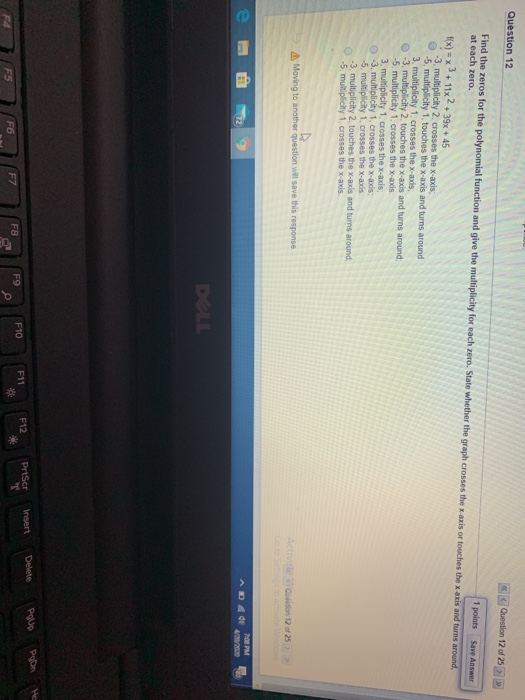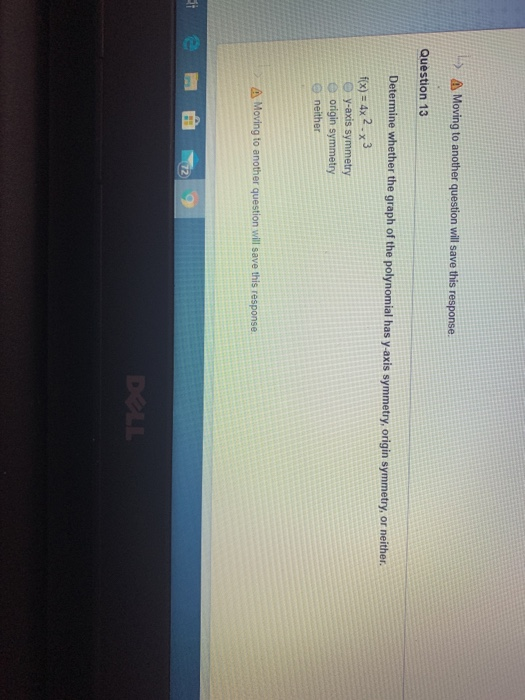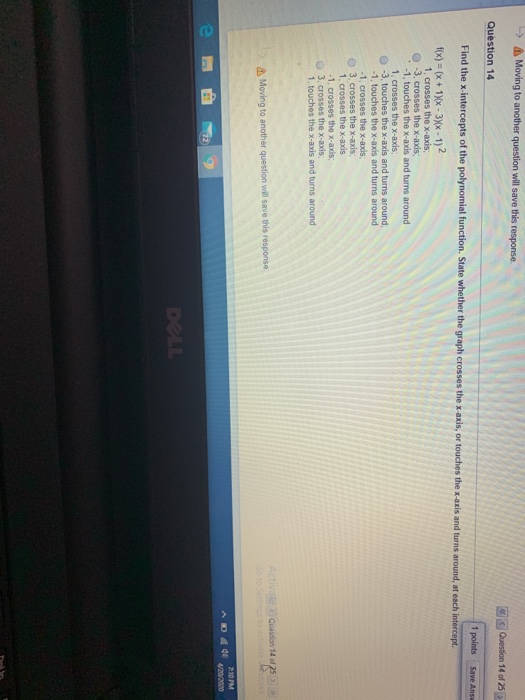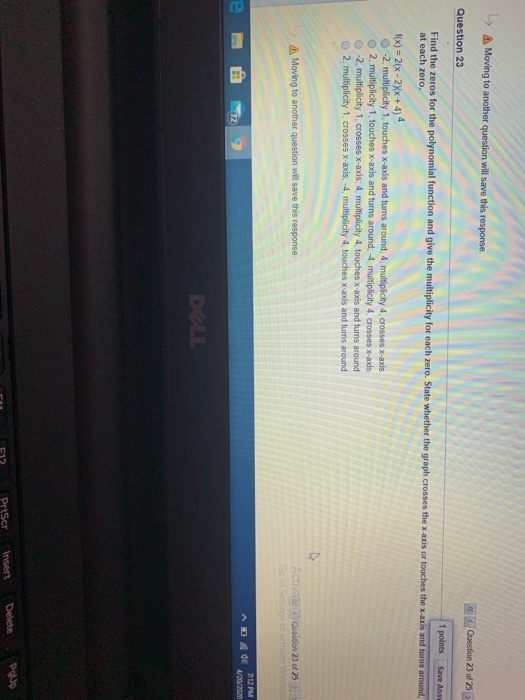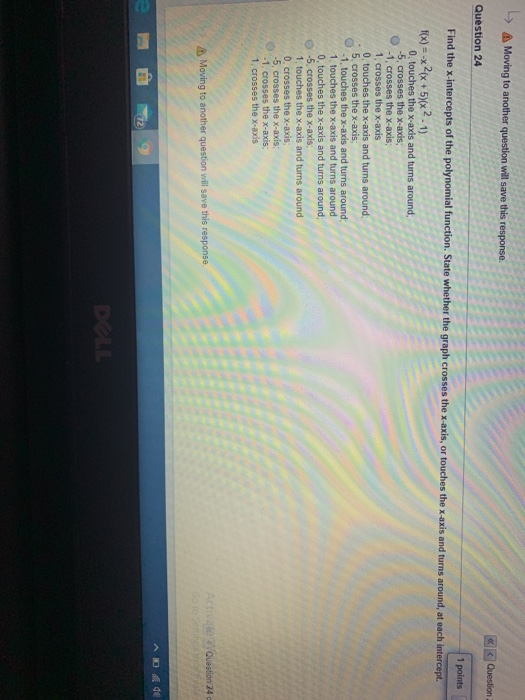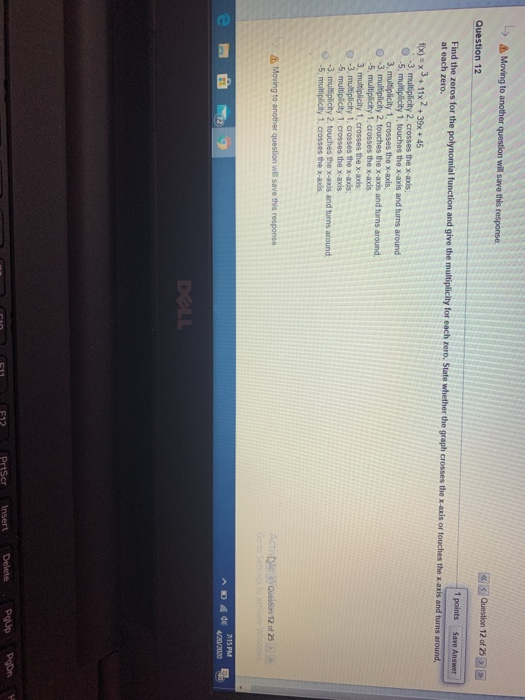Question 12 of 25 > >> Question 12 1 points Save Answer Find the zeros for the polynomial function and give the multiplicity for each zero. State whether the graph crosses the x-axis or touches the x-axis and turns around, at each zero. f(x) = x +11x2 + 3x + 45 3. multiplicity 2. crosses the x-axis -5 multiplicity 1. touches the x-axis and turns around 3. multiplicity 1. crosses the x-ads , multiplicity 2. touches the x-axis and turns around -5 multiplicity 1. crosses the x-axis 3. multiplicity 1. crosses the x-axis 3, multiplicity 1. crosses the x-ads -5 multiplicity 1. crosses the X-axis -3. multiplicity 2. touches the X-ads and turns around 5. multiplicity 1. crosses the x-axis. Moving to another question will save this response Q on 12 of 25 AD 4 4 4 DLL
> Moving to another question will save this response. Question 13 Determine whether the graph of the polynomial has y-axis symmetry, origin symmetry, or neither. f(x) = 4x 2.33 y-axis symmetry e origin symmetry neither Moving to another question will save this response. DALL
-> Moving to another question will save this response. Question 14 of 25 Question 14 1 points Save Ans Find the x-intercepts of the polynomial function. State whether the graph crosses the x-a the x-axis and turns around, at each intercept f(x) = (x + 1)(x - 3)(x - 1) 1. crosses the x-axis; 0 -3. crosses the x-axis; -1, touches the x-axis and turns around 1. Crosses the x-axis, -3, touches the x-axis and turns around -1. touches the x-axis and turns around -1. Crosses the x-axis 3. crosses the X-axis 1. crosses the x-axis -1. Crosses the x-axis, 3. Crosses the x-axis 1. touches the x-axis and turns around Activate Question 14 of 25 Moving to another question will save this response 2:10 PM A D 4 4 /202020
> Moving to another question will save this response. Question 23 of 25 Question 23 1 points Save Ans Find the zeros for the polynomial function and give the multiplicity for each zero. State whether the graph crosses the x-axis or touches the x-axis and turns around, at each zero. f(x) = 2(x-2)(x + 4) 4 -2. multiplicity 1, touches X-axis and turns around. 4. multiplicity 4. crosses x-axis 2. multiplicity 1. touches X-axis and turns around 4. multiplicity 4, crosses x-axis -2, multiplicity 1. crosses x-axis: 4, multiplicity 4, touches x-axis and turns around 2. multiplicity 1, crosses X-axis: -4 multiplicity 4, touches x-axis and turns around > Moving to another question will save this response. Activate Question 23 of 25 COOTED ADA 2:12 PM e @ 729 1/20/2020 1 F12 P PrtScr Insert Delete
-> Moving to another question will save this response Question Question 24 1 points Find the x-intercepts of the polynomial function. State whether the graph crosses the x-axis, or touches the x-axis and turns around, at each intercept f(x) = -x2(x + 5)(x2-1) 0 touches the x-axis and turns around 5. crosses the x-axis: -1, crosses the x-axis 1. crosses the x-axis 0, touches the x-axis and turns around 5. crosses the x-axis: -1. touches the x-axis and turns around; 1. touches the x-axis and turns around 0, touches the X-axis and turns around -5, crosses the x-axis 1. touches the x-axis and turns around 0. crosses the x-axis -5, crosses the x-axis: -1. crosses the x-axis: 1. crosses the x-axis Activate Question 24 Moving to another question will save this response ADA 48
Question 12 of 25 > Moving to another question will save this response Question 12 1 points Save Answer Find the reros for the polynomial function and give the multiplicity for each ro. State whether the graph crosses the axis or touches the axis and turn around at each zero. f(x) = x + 11x2 + 3x + 45 -3, multiplicity 2. Cross the x-x . 5. mu city 1. touches the x-axis and bums around 3. multiplicity 1. crosses the was 3. multiplicity 2. touches the x-axis and turn around -5 municity 1. Coses the x-axis 3. m i dity 1. crosses the xa 3. multiplicity 1. crosses the x-ands -5 multipicity 1. Crosses the ans - multiplicity 2 touches the x- a andums around -5. multiplicity 1. crosses the x-as De Queition 1225 save the response Moving to another question 7:15 PM DUL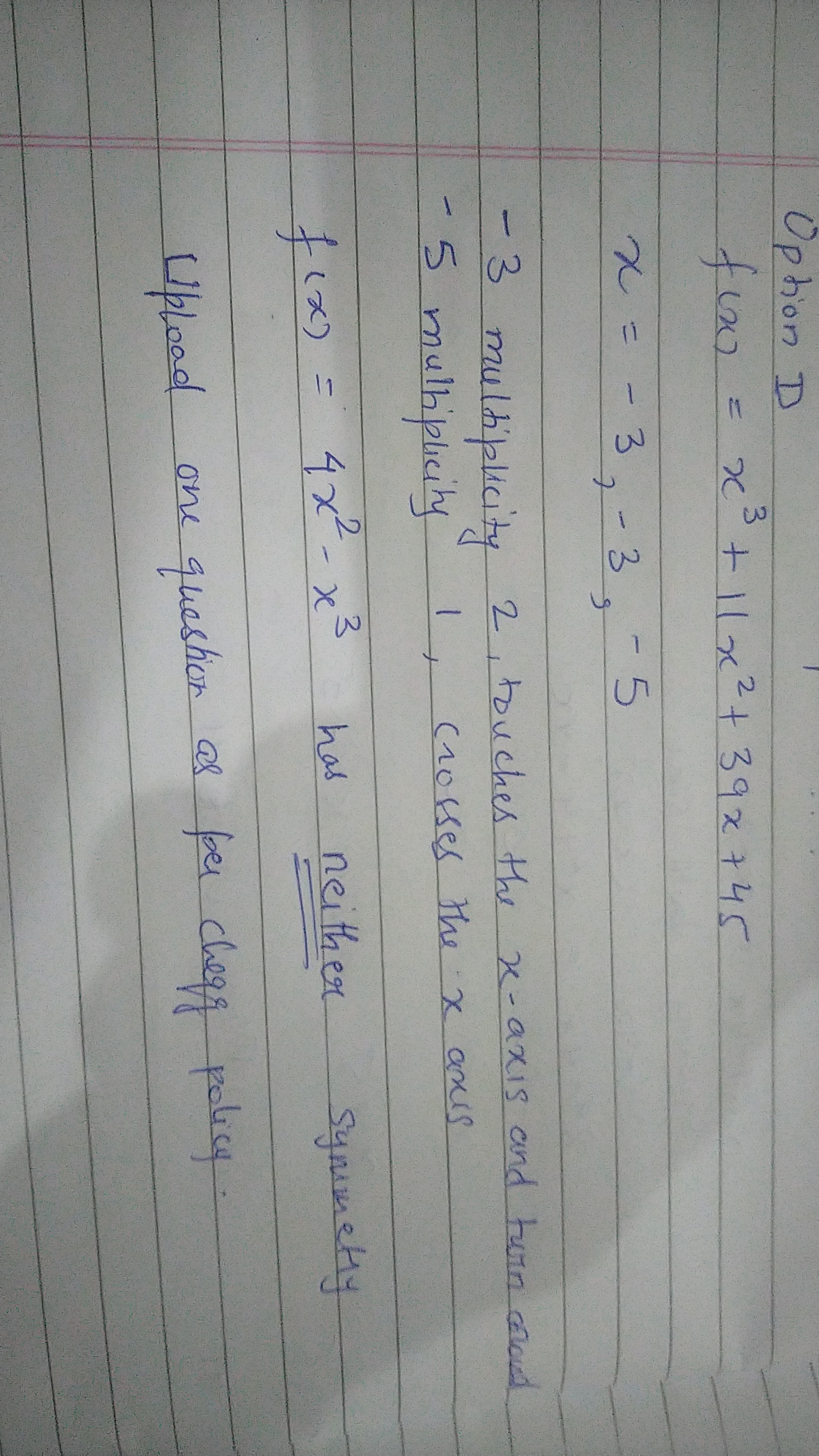#### Earn Coin

Coins can be redeemed for fabulous gifts.

Similar Homework Help Questions
• ### Find the zeros for the given polynomial function and give the multiplicity for each zero. State...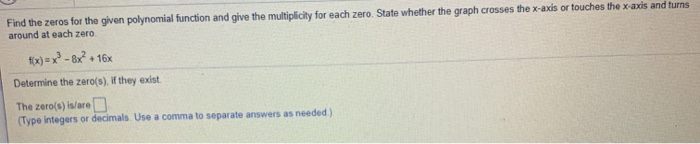Find the zeros for the given polynomial function and give the multiplicity for each zero. State whether the graph crosses the x-axis or touches the x-axis and turns around at each zero fix)=x2-8x? + + 16x Determine the zero(s). If they exist. The zero(s) is/are D (Type integers or decimals. Use a comma to separate answers as needed) Find the zeros for the given polynomial function and give the multiplicity for each zero. State whether the graph crosses the x-axis...

• ### Review for Sections 3.2 and 3.3: 1. [3 pts] Use synthetic division to divide x5 –...

Review for Sections 3.2 and 3.3: 1. [3 pts] Use synthetic division to divide x5 – x4 – 3x3 – 4x2 + 2x – 51 by x – 3. (Show algebraic work.) 2. [4 pts] Solve the equation x3 – 11x2 + 38x – 40 = 0 given that 4 is a zero of f (x) = x3 – 11x2 + 38x – 40. (Show algebraic work.) 3. [5 pts] Answer parts a. – c. below for the given polynomial...

• ### 2.3 2.5

Find the zeros for the polynomial function and give the multiplicity for each zero. State whether the graph crosses the x-axis or touches the x-axis and turns around at each zero.f(x)= -2(x-9)(x+3)2

• ### graph

determine the end behavior of the graph,find the zeros for each polynomial function,and give the multiplicity for each zero.state whether the graph crosses thex-axis,or touches the x-axis and turns around,at each zero.F(x)=3x(x+5)(x+2)^2

• ### Find the x-intercepts of the polynomial function State whether the graph crosses the x-axis, or touches...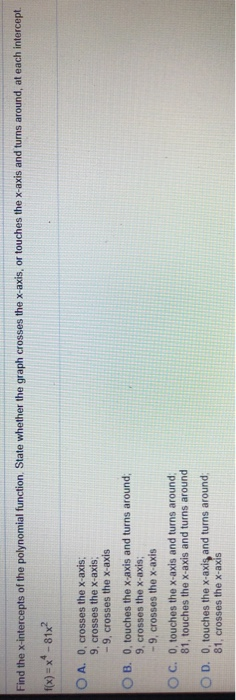Find the x-intercepts of the polynomial function State whether the graph crosses the x-axis, or touches the x-axis and turns around, at each intercept. f(x) = ** -81x? O A. O, crosses the x-axis: 9. crosses the x-axis: -9, crosses the x-axis OB. O, touches the x-axis and turns around; 9, crosses the x-axis; -9, crosses the x-axis OC. 0, touches the x-axis and turns around 81, touches the x-axis and turns around OD. O, touches the x-axis and turns...

• ### Quiz: Quiz 8 3.1, 3.2 This Question: 1 pt In the following exercise, find the coordinates of the ...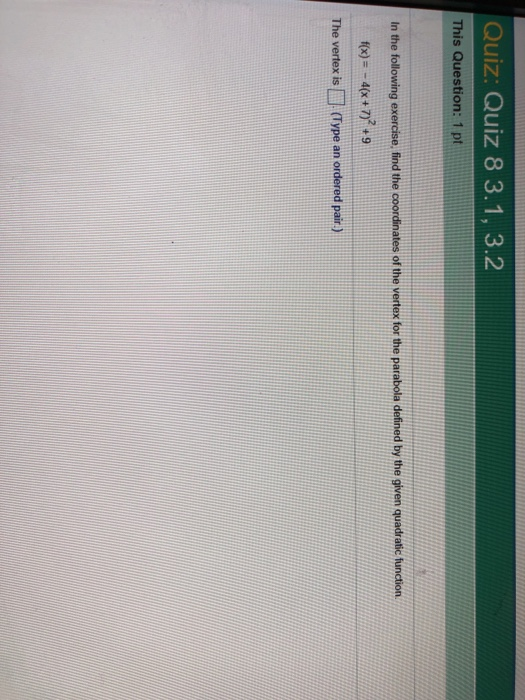Quiz: Quiz 8 3.1, 3.2 This Question: 1 pt In the following exercise, find the coordinates of the vertex for the parabola defined by the given quadratic function. t(x)#-4(x + 7)2 + 9 The vertex is (Type an ordered pair.) 165 SL2 SP 2019 College Algebra DEAN Quiz: Quiz 8 3.1, 3.2 This Question: 1 pt 5 of 12 Find the zeros for the polynomial function and give the multiplicity for each zero. State whether the graph crosses the s-ads...

• ### find the x-intercepts of the polynomial fuction

find the x-intercepts of the polynomial fuction.state weather the graph crosses the x-axis or touches the x-axis and turns around at each intercept. x^5-30x^3+125x=0

• ### Answer parts a.-e. for the function shown below. #x-x3-4x2-x+4 a. Use the leading coefficient tes...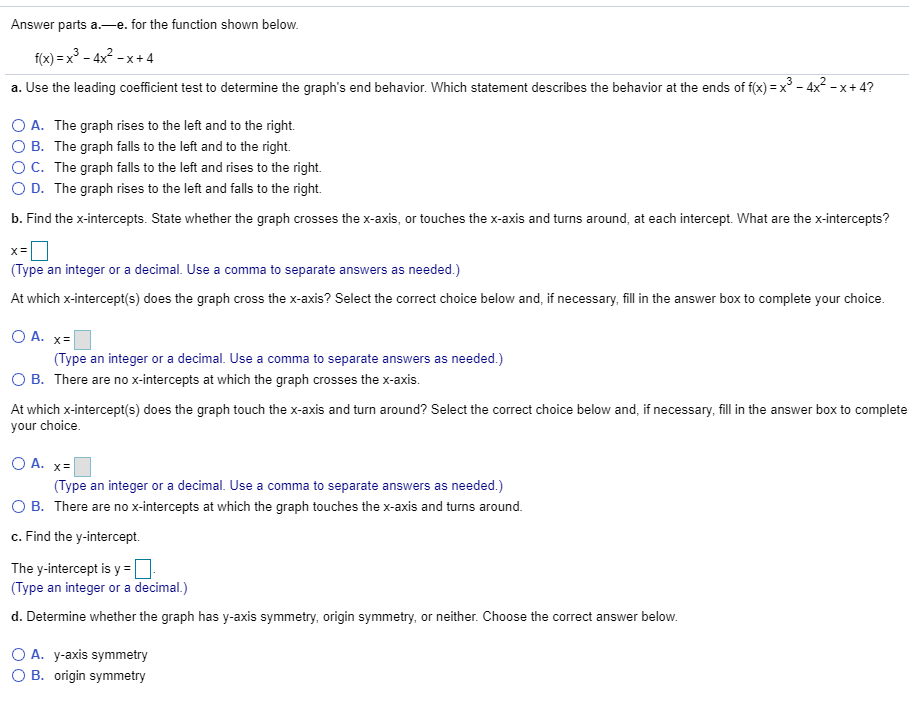Answer parts a.-e. for the function shown below. #x-x3-4x2-x+4 a. Use the leading coefficient test to determine the graph's end behavior. Which statement describes the behavior at the ends of f(x)=x"-4X-x+4? O A. The graph rises to the left and to the right. B. The graph falls to the left and to the right. ° C. The graph falls to the left and rises to the right. D. The graph rises to the left and falls to the right. b....

• ### 1) find the vertex and axis of symmetry of the graph of the function. 2) determine...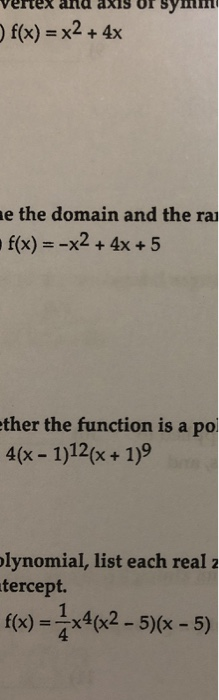1) find the vertex and axis of symmetry of the graph of the function. 2) determine the domain and the range of the function. 3) State whether the function is a polynomial function or not. If it is, give its degree. If it is not, tell why not. 4) For the polynomial, list each real zero and it’s multiplicity. Determine whether the graph crosses or touches the x–axis at each x-intercept. vertex and axIS oF Sm f(x) = x2 +...

• ### Analyze the polynomial function f(x)=x2 + x2 - 20x. Complete parts (a) through (c). (a) Find...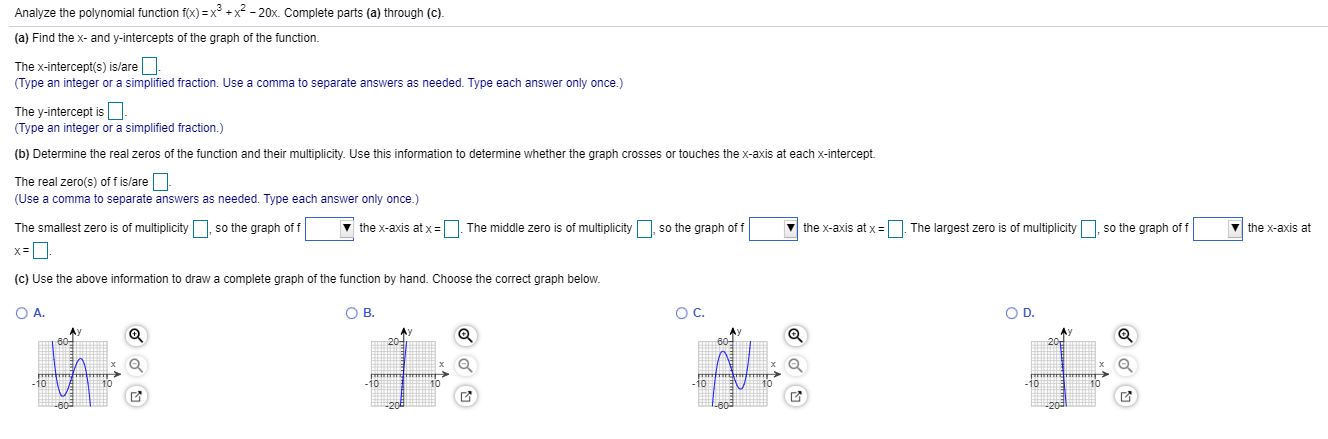Analyze the polynomial function f(x)=x2 + x2 - 20x. Complete parts (a) through (c). (a) Find the x- and y-intercepts of the graph of the function. The x-intercept(s) is/are - (Type an integer or a simplified fraction. Use a comma to separate answers as needed. Type each answer only once.) The y-intercept is - (Type an integer or a simplified fraction.) (b) Determine the real zeros of the function and their multiplicity. Use this information to determine whether the graph...